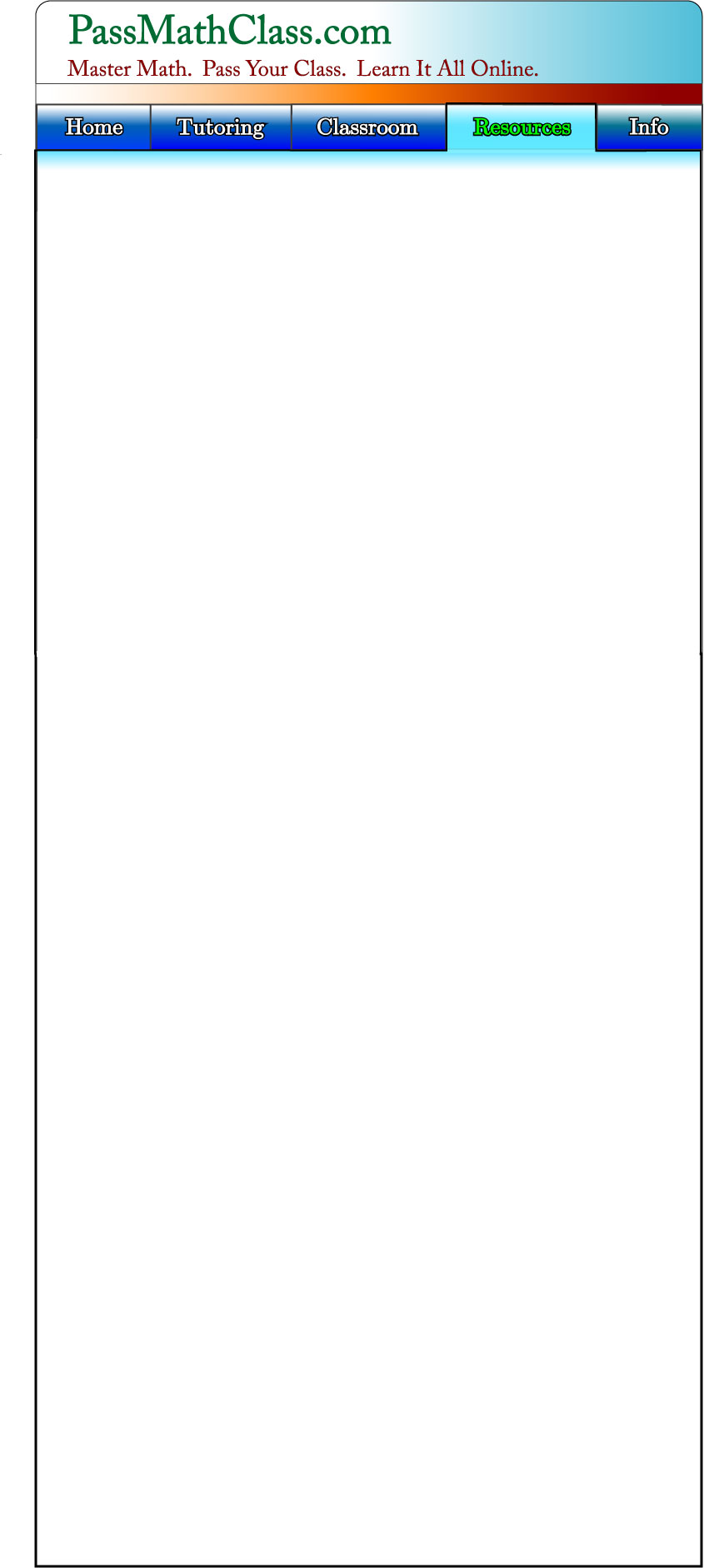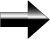Word Problems

Word problems are one of the most difficult parts of algebra for many people.  Once we understand the key concepts of algebra, we must be able to apply them to real examples.  Word problems are one of the most common ways to apply our knowledge.  Like anything in math (or life), solving word problems is easy once you learn how to do it.  We will show you the tricks to solve any word problem.

Solving word problems is a 5-step process.  As you progress, you can cut out or combine many of the steps listed here.  Many of these steps are or will become second-nature to you.

Step 1:  Read The Problem.  Try to get a sense of what the problem is asking.

Step 2:  Re-read the problem for content now.  Identify the key pieces of information given in the problem – determine what exactly the problem is asking you for.

Step 3:  Translate the problem from English to “Math Language”.  Define what your variables are and what they represent.  In other words, write out the equations that you will solve.  After this step, you will have converted the word problem into a normal math problem that you are use to solving.  An easy way to do this is to have a little “English To Math Dictionary” with you while you are solving practice problems.  After you use this method and start to master it, you will naturally begin to translate the words into math automatically.  Click here for the

Step 4:  Solve the equations you wrote in step 3.

Step 5:  Check your work.  Make sure your answer is reasonable and that you didn’t make any mistakes in your setup or algebra.

Proficiency in word problems takes practice.  Learn the basic algebra concepts first, and then learn how to apply them in a word problem.  Turn the word problem into a simple set of equations that you already know how to solve.

Main Algebra Page